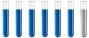## Enthalpy (thermochemistry question)

Chemistry and homework help forum.

Organic Chemistry, Analytical Chemistry, Biochemistry, Physical Chemistry, Computational Chemistry, Theoretical Chemistry, High School Chemistry, Colledge Chemistry and University Chemistry Forum.

Share your chemistry ideas, discuss chemical problems, ask for help with scientific chemistry questions, inspire others by your chemistry vision!

Please feel free to start a scientific chemistry discussion here!

Discuss chemistry homework problems with experts!

Ask for help with chemical questions and help others with your chemistry knowledge!

Moderators: expert, ChenBeier, Xen

Dhamnekar Winod
Sr. Staff MemberPosts: 193
Joined: Sat Nov 21, 2020 10:14 am
Location: Mumbai[Bombay],Maharashtra State,India

### Enthalpy (thermochemistry question)

During a recent winter month in Sheboygan, Wisconsin, it was necessary to obtain 3500 kWh of heat provided by a natural gas furnace with 89% efficiency to keep a small house warm (the efficiency of a gas furnace is the percent of the heat produced by combustion that is transferred into the house).

a)Assume that natural gas is pure methane and determine the volume of natural gas in cubic feet that was required to heat the house. The average temperature of the natural gas was 56 °F; at this temperature and a pressure of 1 atm, natural gas has a density of 0.681 g/L.

b)How many gallons of LPG (liquefied petroleum gas) would be required to replace the natural gas used? Assume the LPG is liquid propane [C3H8: density, 0.5318 g/mL; enthalpy of combustion, 2219 kJ/mol for the formation of CO2(g) and H2O(l)] and the furnace used to burn the LPG has the same efficiency as the gas furnace.

c)What mass of carbon dioxide is produced by combustion of the methane used to heat the house?

d)What mass of water is produced by combustion of the methane used to heat the house?

e)What volume of air is required to provide the oxygen for the combustion of the methane used to heat the house? Air contains 23% oxygen by mass. The average density of air during the month was 1.22 g/L.

f)How many kilowatt–hours (1 kWh = 3.6 × 10⁶ J) of electricity would be required to provide the heat necessary to heat the house? Note electricity is 100% efficient in producing heat inside a house.

g)Although electricity is 100% efficient in producing heat inside a house, production and distribution of electricity is not 100% efficient. The efficiency of production and distribution of electricity produced in a coal-fired power plant is about 40%. A certain type of coal provides 2.26 kWh per pound upon combustion. What mass of this coal in kilograms will be required to produce the electrical energy necessary to heat the house if the efficiency of generation and distribution is 40%?

How to answer a, b, c, d, e, f and g?

What are the answers to a, b, c, d, e, f and g?
Any chemistry help or correct answers to all these questions will be accepted.
Any science consists of the following process. 1) See 2)Hear 3)Smell,if needed 4)Taste, if needed 5)Think 6)Understand 7)Inference 8)Take decision [Believe or disbelieve, useful or useless, true or false, cause or effect, any other criteria]
Dhamnekar Winod
Sr. Staff MemberPosts: 193
Joined: Sat Nov 21, 2020 10:14 am
Location: Mumbai[Bombay],Maharashtra State,India

### Re: Enthalpy (thermochemistry question)

While answering Q(a), it is stated that average temperature at 1 atm pressure was 56°F(13.3333℃).

Now the combustion of one mole of CH4 (methane) releases ∆ H° 890.4 kJ/mol at 25℃(298 K)

My question is at 13.33333℃ (286.48333 K)what would be ∆H of combustion of one mole of CH4 at 1atm pressure?

My attempts to answer all these questions.

a) It was necessary to obtain 12.6 million kJ of heat provided by the combustion of CH4 with 89% efficiency to keep small house warm. Combustion of CH4 at 25°C and 1 atm pressure releases -890.4 kJ/mol(1). But question states average temperature of CH4 was 13.3333°C with density 0.681 g/L at 1 atm pressure.
Assuming (1) and density of CH4, 0.681 g/L the volume of natural gas that was required to heat the house is 13227.84 ft³. Is ths answer correct?

Anawer to b) 139.756 U.S. gallons of LPG would be required to replace the natural gas used. Is this answer correct?

Answer to c) 699.74 kg. of CO2 is produced by a combustion of CH4 used to heat the house. Is this answer correct?

Answer to d) 572.874 kg. of water is produced by a combustion of CH4 used to heat the house. Is this answer correct?

Answer to e) 3626273.45 liters of air is required to provide the oxygen for the combustion of methane used to heat the house. Is this answer correct?

Answer to f) 3500 kWh of electricity would be required to provide the heat necessary to heat the house assuming 100 % efficiency in producing the heat inside the house. Is this answer correct?

Answer to g) 1756.165 kg. of coal will be required to produce the electrical energy necessary to heat the house if the efficiency in generation and distribution of electrical energy is 40%.